# Plotly之高级使用说明2020年3月28日07:28:04

4 5095字阅读16分59秒

## Plotly基础介绍

plotly.py是提供了一个python使用Plotly.js(JavaScript library)这个的接口. 在Plotly.js中, 一个figure与一个JSON文件是对应的. 我们可以看下面的一个例子, JSON文件是如何与Figure对应的.

1. fig = {
2.     "data":[{'type': 'bar',
3.             'x': [1,2,3],
4.             'y': [1,3,2]}],
5.     "layout": {"title": {"text": "A Bar Chart"}}
6. }
7. import plotly.io as pio
8. pio.show(fig)

• 在data中我们定义了要绘制图像的种类和里面的数值.
• 在layout中我们可以定义图像的布局, 例如标题等.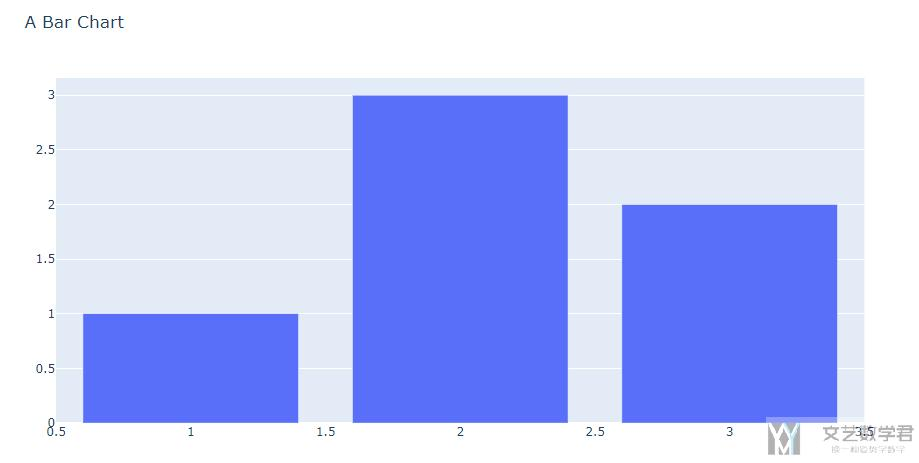### 一些准备

1. import plotly.express as px
2. import plotly.graph_objects as go

### Plotly中的graph objects

• 使用Graph objects报错更加详细, 如果你有地方参数有问题, 可以有更加详细的报错信息.
• 对于各个属性的修改, 我们可以使用fig['layout']或是fig.layout进行修改.

1. fig = go.Figure(
2.     data=[go.Bar(x=[1, 2, 3], y=[1, 3, 2])],
3.     layout=go.Layout(
4.         title=go.layout.Title(text="A Bar Chart")
5.     )
6. )
7. fig.show()1. fig.to_json()
2. fig.to_dict()

### Plotly中的Plotly Express

Plotly Express是提供了一种更加高级的API来创建graph object. 我们还是使用上面的绘制bar的例子来进行说明.

1. # 创建数据集
2. data_test = pd.DataFrame([[1,1],[2,3],[3,2]], columns=['x','y'])
3. # 进行绘图
4. fig = px.bar(data_test, x='x', y='y')
5. fig.update_layout(title="A Bar Chart") # 这个时候也是可以更新图像的标题
6. fig.show()## Plotly更新图像

1. fig = go.Figure()
2. fig.add_trace(go.Bar(x=[1, 2, 3], y=[1, 3, 2]))
4. fig.show()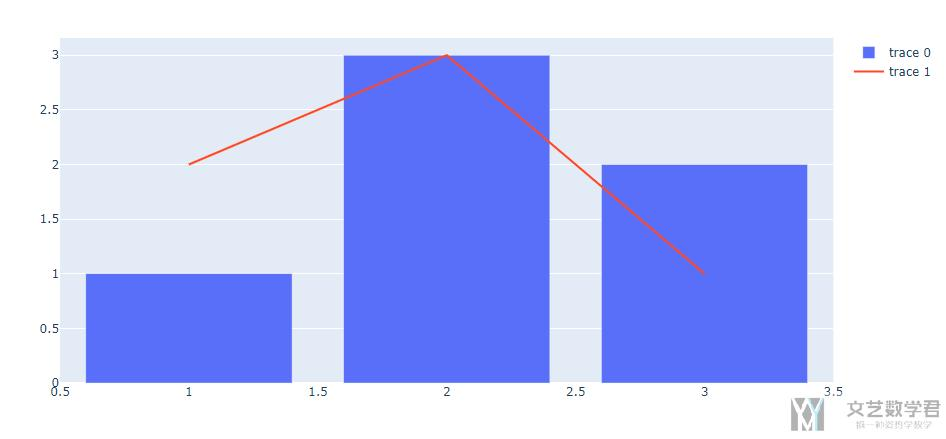#### 与Plotly Express图形组合

1. df = px.data.iris()
2. fig = px.scatter(df, x="sepal_width", y="sepal_length", color="species")
4.     go.Scatter(
5.         x=[2, 4],
6.         y=[4, 8],
7.         mode="lines",
8.         name='Regression',
9.         line=go.scatter.Line(color="gray"),
10.         showlegend=True)
11. )
12. fig.show()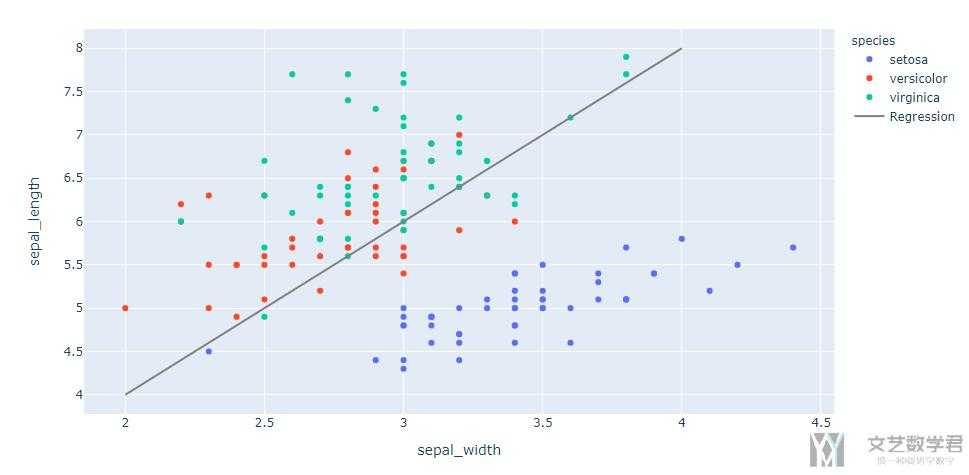1. fig = go.Figure()
3. fig.show()

### 更新图像布局-update_layout

1. fig = go.Figure(data=go.Bar(x=[1, 2, 3], y=[1, 3, 2]))
2. fig.update_layout(title_text="A Bar Chart",
3.                   title_font_size=30)
4. fig.show()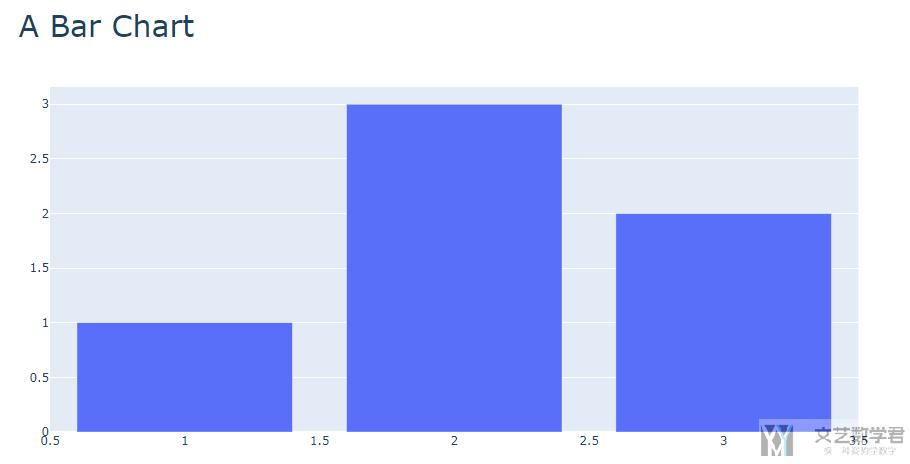1. fig.update_layout(xaxis_type="log")

### 更新坐标轴-update_xaxes

1. df = px.data.iris()
2. fig = px.scatter(df, x="sepal_width", y="sepal_length", color="species", facet_col="species")
3. fig.update_xaxes(showgrid=False)
4. fig.show()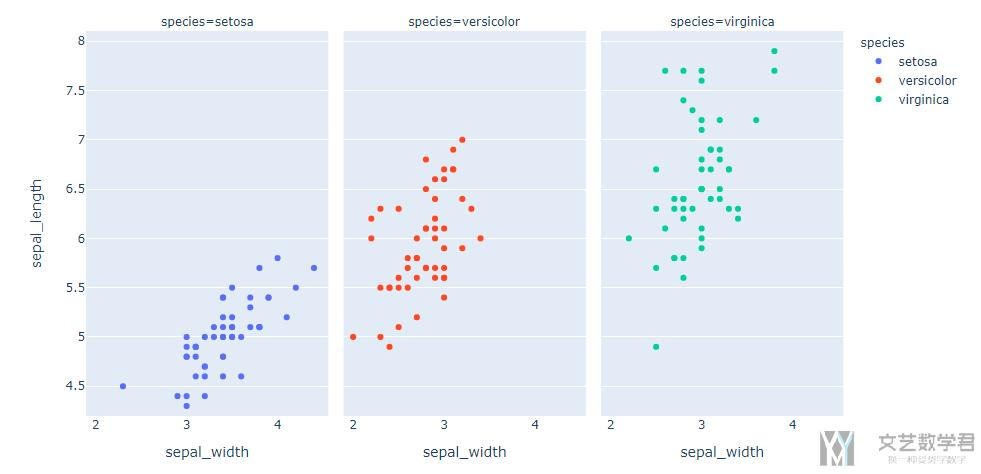## Plotly子图的绘制

### 基本的子图绘制

1. from plotly.subplots import make_subplots

1. fig = make_subplots(rows=1, cols=2)
2. fig.add_trace(go.Scatter(y=[4, 2, 1], mode="lines"), row=1, col=1)
3. fig.add_trace(go.Bar(y=[2, 1, 3]), row=1, col=2)
4. fig.show()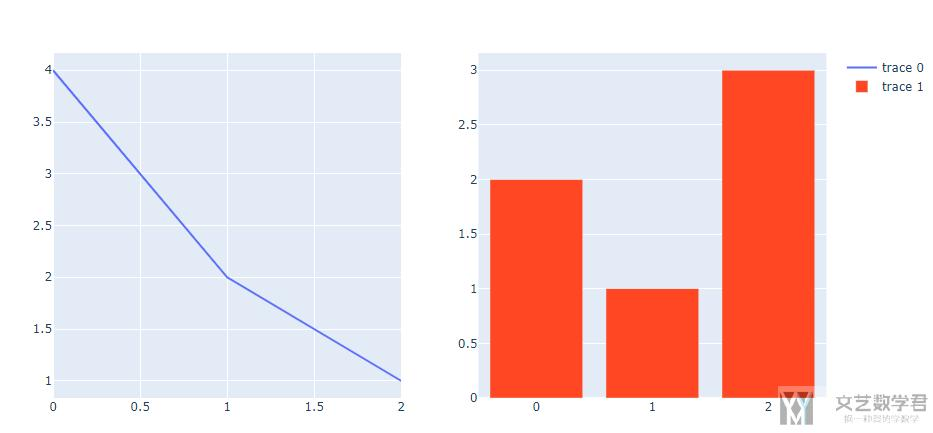### 子图中包含多幅图像

1. fig = make_subplots(rows=1, cols=2)
2. # 1,1
3. fig.add_trace(go.Scatter(y=[4, 2, 1], mode="lines"), row=1, col=1)
4. fig.add_trace(go.Scatter(y=[3, 4, 2], mode="lines"), row=1, col=1)
5. # 1, 2
6. fig.add_trace(go.Bar(y=[2, 1, 3]), row=1, col=2)
7. fig.show()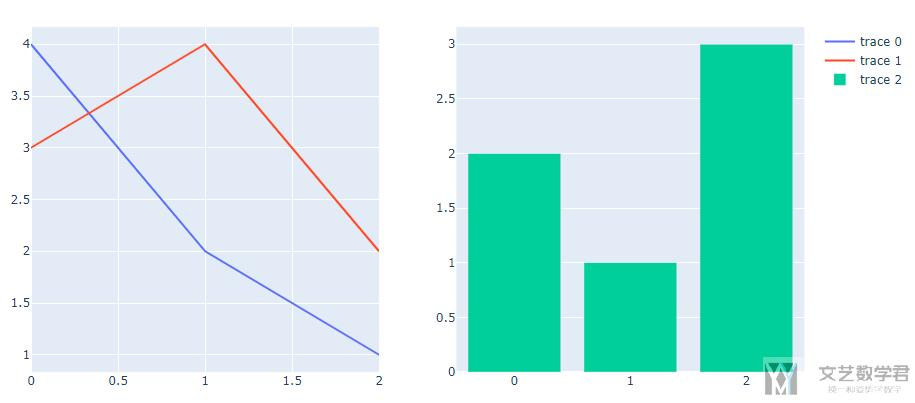### 关于多幅图像同时更新-update_traces

1. fig = make_subplots(rows=2, cols=2)
2. # 1, 1
4.                 marker=dict(size=20, color="LightSeaGreen"),
5.                 name="a", row=1, col=1)
7.             marker=dict(color="MediumPurple"),
8.             name="b", row=1, col=1)
9. # 1, 2
11.                 marker=dict(size=20, color="MediumPurple"),
12.                 name="c", row=1, col=2)
14.             marker=dict(color="LightSeaGreen"),
15.             name="d", row=1, col=2)
16. # 2, 1
18.                 marker=dict(size=20, color="MediumPurple"),
19.                 name="e", row=2, col=1)
21.             marker=dict(color="LightSeaGreen"),
22.             name="f", row=2, col=1)
23. # 2, 2
25.                 marker=dict(size=20, color="MediumPurple"),
26.                 name="g", row=2, col=2)
28.             marker=dict(color="LightSeaGreen"),
29.             name="h", row=2, col=2)
30. fig.show()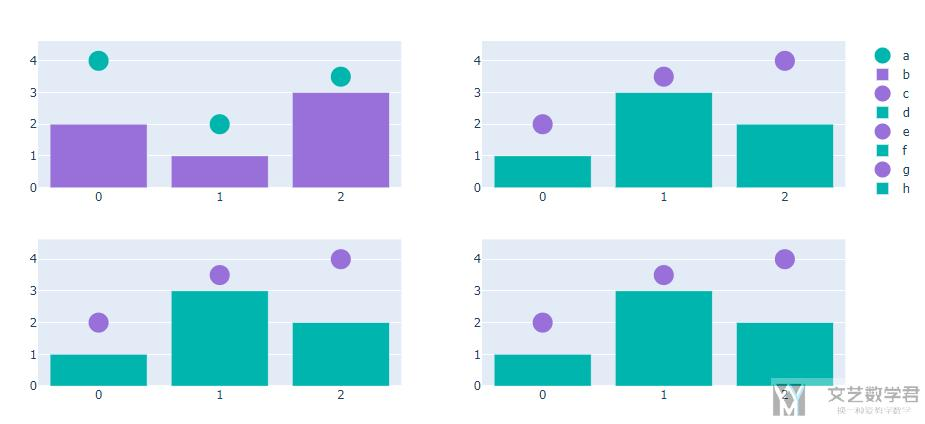1. # update
2. fig.update_traces(marker=dict(color="RoyalBlue"),
3.                  selector=dict(type="bar"),
4.                  row=1)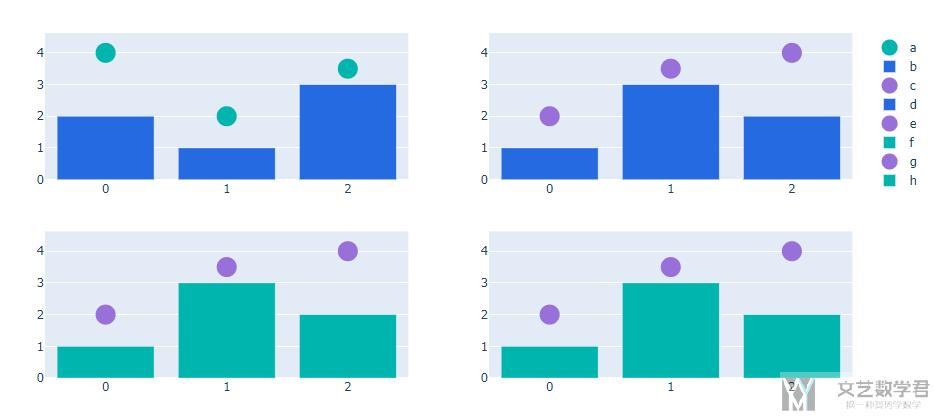• 微信公众号
• 关注微信公众号
•• QQ群
• 我们的QQ群号
•• 本文由 发表于 2020年3月28日07:28:04
• 转载请务必保留本文链接：https://mathpretty.com/11954.html